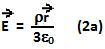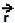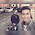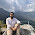## Friday, May 7, 2010

### Irodov Problem 3.28This problem is an excellent illustration of the superposition principle of electrostatic. The basic idea is to understand that the electric field due to the sphere with a displaced cavity can be represented as the sum of electric fields due to a solid sphere of positive charge densityand another displaced sphere with negative charge density of(the negative and positive charge densities cancel each other in the volume of the displaced sphere thus creating the displaced cavity).

Now let us compute the electric filed inside a uniformly charged sphere at a distance r from the center. As in case of problems 3.25-3.27, we can compute this electric field using a Spherical Gaussian surface concentric with the original sphere. The total charge contained within the Gaussian surface will be,Now using Gauss Law we can write,Further, since the electric field vector will in a direction radially outwards (2) can be written in vector notation as,A point located atwill be at a locationas seen from the center of the sphere displaced such that its center lies atwith respect to the center of the original sphere. Consequently, the electric fields due to each of the spheres based on (2a) will be,Hence, the net electric field inside the cavity will be,1.This comment has been removed by the author.

2.what will d trajectory of an electron be if kept on the inner surface of the cavity?

1.May be its opposite to the direction of vec. a..

3.Would someone please correct my line of reasoning? If we were to draw a spherical Gaussian surface centred at point 'a' with radius 'r-a' passing through the point where we are computing the field strength, wouldn't it be zero? Since the cavity encloses no net charge?

1.Electric field here due to cavity would be zero,but from the sphere there will be some electric field and would be non uniform in the case so can't be calculated using gauss law

2.This comment has been removed by the author.

3.as per my intuition this is not a metal sphere.. so there can be electric lines inside the cavity

4.This comment has been removed by the author.

4.Cours u please explain how do u got radius officiel cavity as r-a ??